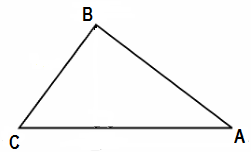Guru

# Tick the correct answer and justify: In ΔABC, AB = 6√3 cm, AC = 12 cm and BC = 6 cm. The angle B is: (A) 120° (B) 60° (C) 90° (D) 45° Q.17

• 0

What is the best solution for the question of Chapter of Triangles of exercise 6.5 of ncert 10th math, of question no.17, Please give me the best way to solve this problem in easy way, Tick the correct answer and justify: In ΔABC, AB = 6√3 cm, AC = 12 cm and BC = 6 cm. The angle B is: (A) 120° (B) 60° (C) 90° (D) 45°

Share

1. Given, in ΔABC, AB = 6√3 cm, AC = 12 cm and BC = 6 cm.We can observe that,

AB2 = 108

AC2 = 144

And, BC2 = 36

AB2 + BC2 = AC2

The given triangle, ΔABC, is satisfying Pythagoras theorem.

Therefore, the triangle is a right triangle, right-angled at B.

∴ ∠B = 90°

Hence, the correct answer is (C).

• 0